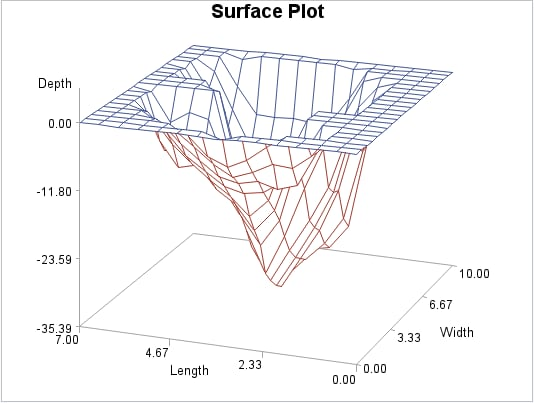# Overview: G3D Procedure

The G3D procedure enables you to produce three-dimensional surface plots and scatter plots.

## Surface Plots

Surface plots represent the shape of the surface that is described by the values of three variables, X, Y, and Z. The values of the X and Y variables are plotted to form a horizontal plane. The values of the Z variable, create a vertical axis that is perpendicular to the X-Y plane. Combined, these three axes, form a three-dimensional surface.
The surface plot in the following figure displays various depths of a lake. The dimensions of the lake are plotted on the X-Y axes. The Z variable is plotted as the third dimension. The coordinates of each point correspond to the values of the three numeric variable values in an observation from the selected input data set.
G3D Surface PlotWith the PLOT statement, you can do the following actions:
• show the three-dimensional shape of your data (useful for examining data trends).
• change the data ranges that are displayed.
• rotate and tilt the plot to enhance viewing angles.
• customize the axes.
The program for this figure is featured in Generating A Surface Plot. For more information about producing surface plots, see the PLOT Statement.

## Scatter Plots

Scatter plots represent the data as points. As with surface plots, the values of the X and Y variables are plotted to form a horizontal plane. The values of the Z variable create a vertical axis that is perpendicular to the X-Y horizontal plane. The values of the Z variable are represented as individual symbols. By default, these symbols are connected to the horizontal plane with lines, referred to as needles.
G3D Scatter PlotWith the SCATTER statement, you can do the following actions:
• change the symbols used to represent your data points
• categorize your data with colors, shapes, sizes
• change the data ranges that are displayed
• rotate and tilt the plot to enhance the viewing angles
• customize the axes